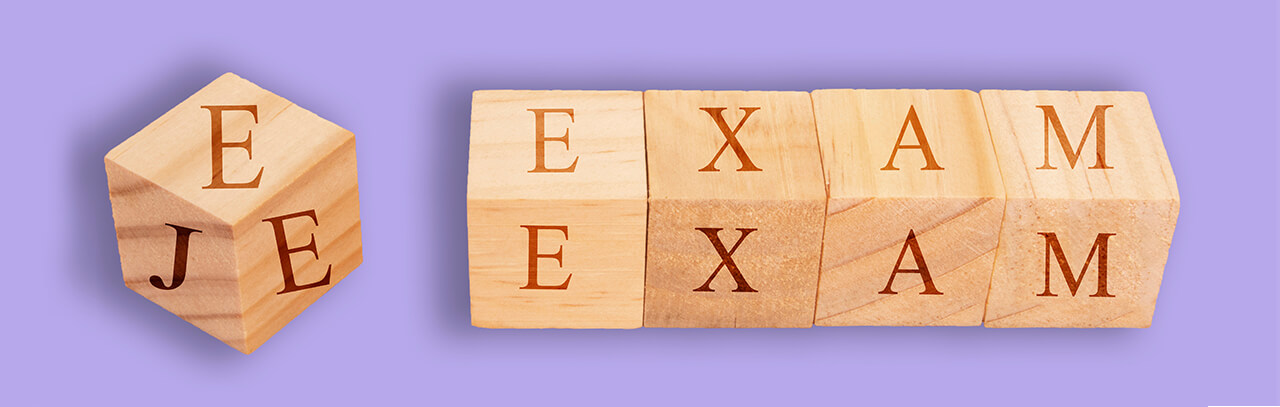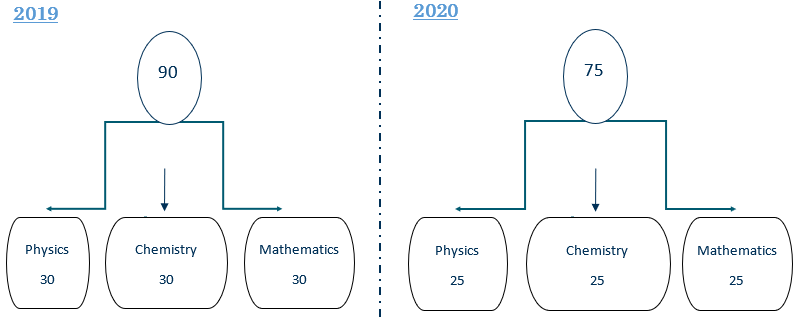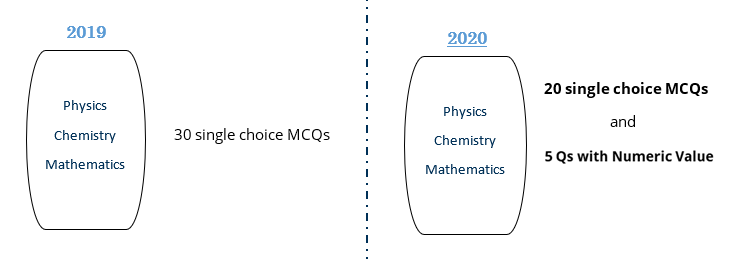# What to expect in JEE Main January 2020

Changes in JEE Main 2020

This year, NTA has revised the JEE Main 2020 exam pattern As per the revised JEE Main exam pattern 2020, the question booklet of BE/B.Tech., will have a total of 75 questions instead of 90 questions. These questions will be equally distributed among Physics, Chemistry, and Mathematics, each consisting of 25 questions. Out of the total questions in JEE Main 2020 Paper 1, each subject will have 20 objective type multiple choice questions along with 5 numerical based questions.Here are a few things we must know about the changes.

1. Total questions (75 questions) – From now on, there will be 75 questions instead of 90 questions with 25 each from Physics, Chemistry, and Mathematics.

Number of Questions:2. Type of questions (60 MCQs and 15 numerical types) – From now on, out of total 75 questions, 60 are going to be MCQ type – 20 each from Physics, Chemistry, and Mathematics and 15 questions are going to be numerical based – 5 each from Physics, Chemistry, and Mathematics.

Type of Questions:3. Marking Scheme (No negative marking in numerical questions) – This is a huge advantage from these changes. There is NO negative marking in the 15 numerical based questions. So, when you provide an answer for numerical type questions, if you get it right, you will get (+ 4) marks and even if you don’t get it right, it still wouldn’t affect i.e., no negative marks (- 0 marks).

4. Total marks (300 marks instead of 360) – From now on, there is going to be a total of 75 questions and each question is still going to be of 4 marks. Hence, students will be marked from 300 marks instead of 360 marks.

Looking at these changes, one can observe that there are going to be some advantages for the students. Here are some of them:

1. More minutes per questions – Although the number of questions has been reduced, the time length is still the same. Therefore, students will get more time per answer as compared to earlier. There are going to be 75 questions instead of 90 questions and time length is still of 3 hours. The students will get 2.4 minutes per question to solve, instead of 2 minutes.

2. No negative marking makes it easier to attempt – There is NO negative marking in the 15 numerical based questions. So, even if you aren’t too sure about the answers, you can still provide an answer you can think of, as now you don’t have to worry about the negative marking in numerical type questions. Basically, if you have the idea about the question and you are stuck while solving it, you can still attempt it by providing a possible correct answer.